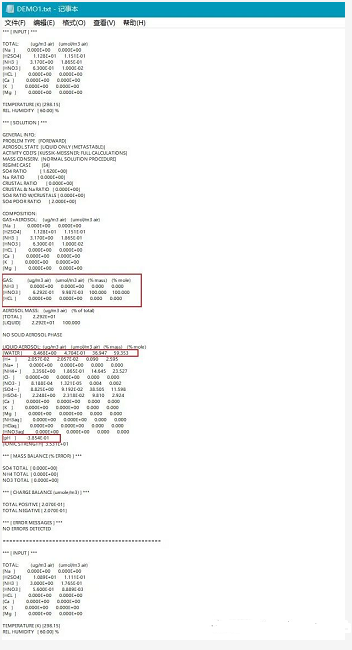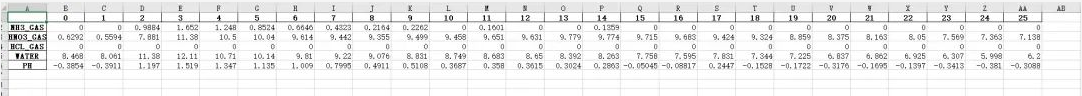2022
05-11

# python如何读取txt文件中满足要求的数据(python如何读取txt文件内容)01txt文档组成及目标数据

txt文档中共分为26部分，每部分以 *** [ INPUT ] ***为起始行，以====为结束行。我的目标数据为如下红框中所标注的第二列数据。可以发现目标数据的位置存在着固定的规律，即在GAS: 所在行的下三行和LIQUID AEROSOL: 所在行的下一行和后15行。目标数据有着固定的规律，便可以使用python编程来自动地提取整个txt文档中的目标数据。02目标数据及格式03代码实现

```import pandas as pd
NH3_GAS = []
HNO3_GAS = []
HCL_GAS = []
WATER = []
PH =[]
with open("DEMO1.txt", "r") as f:
for index,item in enumerate(lines): # 对txt每行内容进行索引赋值
if 'GAS:' in item:
NH3_GAS_temp = lines[index+1].strip('\n').split(' ') # 提取出目标行中的目标数据
NH3_GAS_temp = [x for x in NH3_GAS_temp if x !='']
NH3_GAS.append(NH3_GAS_temp)
HNO3_GAS_temp = lines[index + 2].strip('\n').split(' ')
HNO3_GAS_temp = [x for x in HNO3_GAS_temp if x != '']
HNO3_GAS.append(HNO3_GAS_temp)
HCL_GAS_temp = lines[index + 3].strip('\n').split(' ')
HCL_GAS_temp = [x for x in HCL_GAS_temp if x != '']
HCL_GAS.append(HCL_GAS_temp)

if 'LIQUID AEROSOL:' in item:
WATER_temp = lines[index+1].strip('\n').split(' ')
WATER_temp = [x for x in WATER_temp if x != '']
WATER.append(WATER_temp)
PH_temp = lines[index+15].strip('\n').split(' ')
PH_temp = [x for x in PH_temp if x !='']
PH.append(PH_temp)

data = pd.DataFrame({'NH3_GAS':NH3_GAS,'HNO3_GAS':HNO3_GAS,'HCL_GAS':HCL_GAS,'WATER':WATER,'PH':PH},dtype='float32')
data.T.to_excel('run1.xlsx')
print(data.T)```